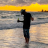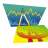P

#### pcex.antiuno

##### New member
Hi Everyone, I'm learning to use ThinkScript and I have a question.

Here is the thing, I made a script in a study with many variables like this (is just an example):

Code:
``````script TestScript{
input num = 5;
def Var1 = num + 1;
def Var2 = num + 2;
plot Var3 = Var1 + Var2;
}``````

and I use It forward like this:

Code:
``````def testNumber1 = 20;
def testNumber2 = 30;

def test11 = TestScript(testNumber1).Var1;
def test12 = TestScript(testNumber1).Var2;
plot test13 = TestScript(testNumber1).Var3;

def test21 = TestScript(testNumber2).Var1;
def test22 = TestScript(testNumber2).Var2;
plot test23 = TestScript(testNumber2).Var3;``````

My question is: Every time I call TestScript(something).someVariable; script calculations are made them again?

Is there a way to call the script just once ? Someway like this (I try it and doesnt work):

Code:
``````def testScript1 = TestScript(testNumber1);

def test11 = testScript1.Var1;
def test12 = testScript1.Var2;
plot test13 = testScript1.Var3;

def testScript2 = TestScript(testNumber2);

def test21 = testScript2.Var1;
def test22 = testScript2.Var2;
plot test23 = testScript2.Var3;``````

I hope someone can help me.

EDIT: I wrote wrong the tittle. Is "Question about scripts references"

S

#### Saylarr

##### Member
@pcex.antiuno Anyone who knows or can answer this? I am also interested in the answer, to use it in a study that I am doing.##### Well-known member
VIP
@pcex.antiuno

The easiest way to run only once would be to define and check with a counter variable... Increment the counter the first time the script runs and then check for the result on subsequent iterations... Subsequent iterations would read the "1" from the first iteration and store it again... There are examples of counter code here in the forums... If you need further help let us know...

P

#### pcex.antiuno

##### New member

Thanks but isn't the answer that I'm looking for. I'd like to use some code like the examples I described before.##### Well-known member
VIP
I gave advice on how to make it only calculate one time as you asked... If you know of a better way then go for it... As I see it, you want the script to run until it meets your criteria just once... How else would you propose that...???

Storm knocked out the power so I'm on my phone which is the wrong tool for the job, other than a bit of reading... I'll check back once power is restored...

P

#### pcex.antiuno

##### New member
I just wanna do something like this example (obviously doesn't work):

Code:
``````def testScript1 = TestScript(testNumber1);

def test11 = testScript1.Var1;
def test12 = testScript1.Var2;
plot test13 = testScript1.Var3;

def testScript2 = TestScript(testNumber2);

def test21 = testScript2.Var1;
def test22 = testScript2.Var2;
plot test23 = testScript2.Var3;``````

Like other programming languages, like this was an object or something like that.
I'd like recalculate if It's necesary but keep the script in one variable and call scripts variables with that. Sorry, I dont know if I'm explain myself.My Question is off topic if there is a topic, Does anyone like P H O N K? Questions 0Site Preferences Question Questions 9